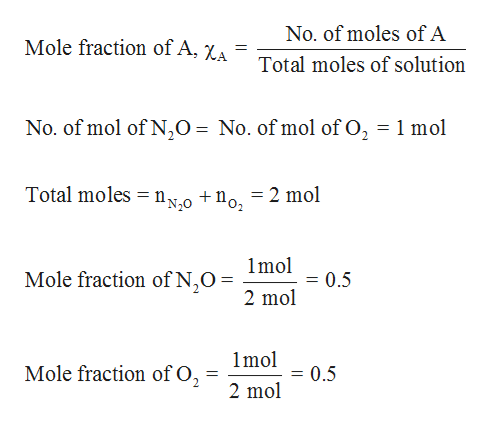# A 1:1 mixture by moles of nitrous oxide and oxygen is often used as a sedative in dentistry. If the total pressure of this mixture in a cylinder is 2.5 atm, what is the partial pressure of each gas? STEP BY STEP WOULD BE APPRECIATED

Question
297 views

A 1:1 mixture by moles of nitrous oxide and oxygen is often used as a sedative in dentistry. If the total pressure of this mixture in a cylinder is 2.5 atm, what is the partial pressure of each gas?

STEP BY STEP WOULD BE APPRECIATED

check_circle

Step 1

Mole fraction of nitrous oxide (N2O) and Oxygen (O2...help_outlineImage TranscriptioncloseNo. of moles of A Mole fraction of A, XA Total moles of solution No. of mol of N20 = No. of mol of O2 1 mol Total moles n0 +no, = 2 mol 1mol Mole fraction of N,O 0.5 2 mol 1mol Mole fraction of O2 0.5 11 2 mol fullscreen

### Want to see the full answer?

See Solution

#### Want to see this answer and more?

Solutions are written by subject experts who are available 24/7. Questions are typically answered within 1 hour.*

See Solution
*Response times may vary by subject and question.
Tagged in

### Gas laws